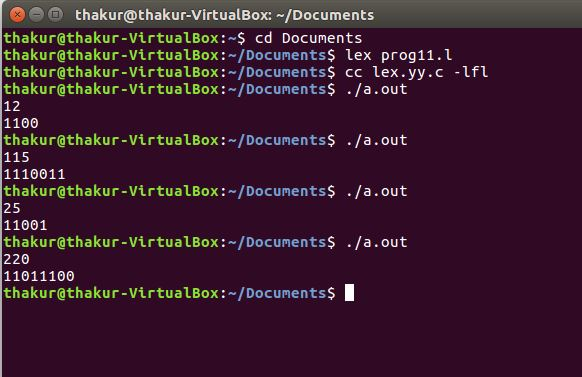Related Articles

# Lex program for Decimal to Binary Conversion

• Difficulty Level : Medium
• Last Updated : 02 May, 2019

Problem: Write a Lex program for Decimal to Binary conversion.

Explanation:
FLEX (Fast Lexical Analyzer Generator) is a tool/computer program for generating lexical analyzers (scanners or lexers) written by Vern Paxson in C around 1987. Lex reads an input stream specifying the lexical analyzer and outputs source code implementing the lexer in the C programming language. The function yylex() is the main flex function which runs the Rule Section.

Examples:

```Input: 12
Output: 1100

Input: 115
Output: 1110011

Input: 25
Output: 11001

Input: 220
Output: 11011100 ```

Implementation:

 `/* Lex program for decimal to binary conversion */`` ` `%{``  ``/* Definition section */``  ``#include``  ``int` `num, r, b=0, p=1;``%}`` ` `DIGIT [0-9]``/* Rule Section */``%%``{DIGIT}+   { num=``atoi``(yytext);``             ``while` `(num > 0)``             ``{``              ``r= num % 2;``              ``b+= r*p;``              ``p*= 10;``              ``num/= 2;``             ``}``              ``printf``(``"%d"``, b);``            ``}``.|\n    ECHO;``        ` `%%`` ` `// driver code ``int` `main()``{``    ``yylex();``    ``return` `0;``}      `

Output:Attention reader! Don’t stop learning now. Get hold of all the important CS Theory concepts for SDE interviews with the CS Theory Course at a student-friendly price and become industry ready.

My Personal Notes arrow_drop_up